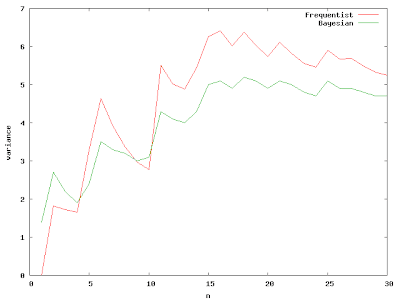## Sunday, October 26, 2008

### Simple Bayes

I like Bayes theorem, it's really useful. The most intuitive and accessible explanation I've found of using Bayes theorem to solve a problem is in Russell and Norvig's classic, Chapter 20 (pdf) (I just own the first edition, the second edition looks even better).

The initial example they give is about pulling different flavoured candy out of a sack (remember the balls and urn from your basic stats?). They also provide a really good discussion showing how standard least-squares regression is a special case of maximum-likelihood for when the data are generated by a process with Gaussian noise of fixed variance.

Their first example is for estimating parameters in a discrete distribution of candy, but we can apply the same math to estimating the variance of a continuous distribution. Estimating variance is important, lots of times in industrial or business settings the variance of a thing matters as much or more than the average, just check-out all of the press those Six Sigma guys get. That's because it gives us insight into our risk. It helps us answer questions like, "What's our probability of success?" And maybe, if we're lucky, "What things is that probability sensitive to?"

Bayes theorem is a very simple equation:Where P(h) is the prior probability of the hypothesis, P(d|h) is the likelihood of the data given the hypothesis, and P(h|d) is the posterior probability of the hypothesis given the data.

Octave has plenty of useful built-in functions that make it easy to play around with some Bayesian estimation. We'll set up a prior distribution for what we believe our variance to be with chi2pdf(x,4), which gives us a Chi-squared distribution with 4 degrees of freedom. We can draw a random sample from a normal distribution with the normrnd() function, and we'll use 5 as our "true" variance. That way we can see how our Bayesian and our standard frequentist estimates of the variance converge on the right answer. The standard estimate of variance is just var(d), where d is the data vector.

The likelihood part of Bayes theorem is:

% likelihood( d | M ) = PI_i likelihood(d_i, M_j)
for j=1:length(x)
lklhd(j) = prod( normpdf( d(1:i), 0, sqrt( x(j) ) ) );
endfor
lklhd = lklhd/trapz(x,lklhd); % normalize it

Then the posterior distribution is:

% posterior( M | d ) = prior( M ) * likelihood( d | M )
post_p = prior_var .* lklhd;
post_p = post_p/trapz(x,post_p); % normalize it

Both of the estimates of the variance converge on the true answer as n approaches infinity. If you have a good prior, the Bayesian estimate is especially useful when n is small.It's interesting to watch how the posterior distribution changes as we add more samples from the true distribution.The great thing about Bayes theorem is that it provides a continuous bridge from what we think we know to reality. It allows us to build up evidence and describe our knowledge in a consistent way. It's based on the fundamentals of basic probability and was all set down in a few pages by a nonconformist Presbyterian minister and published after his death in 1763.

Octave file for the above calculations: simple_bayes.m

1.2.